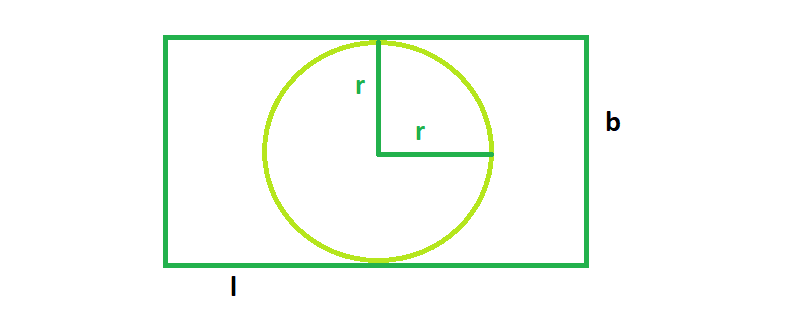# The biggest possible circle that can be inscribed in a rectangle

Given a rectangle of length l & breadth b, we have to find the largest cricle that can be inscribed in the rectangle.
Examples:

```Input  : l = 4, b = 8
Output : 12.56

Input  : l = 16 b = 6
Output : 28.26
```

## Recommended: Please try your approach on {IDE} first, before moving on to the solution.From the figure, we can see, the biggest circle that could be inscribed in the rectangle will have radius always equal to the half of the shorter side of the rectangle. So from the figure,

Area, A = π * (r^2)

## C++

 `// C++ Program to find the biggest circle ` `// which can be inscribed  within the rectangle ` `#include ` `using` `namespace` `std; ` ` `  `// Function to find the area ` `// of the biggest circle ` `float` `circlearea(``float` `l, ``float` `b) ` `{ ` ` `  `    ``// the length and breadth cannot be negative ` `    ``if` `(l < 0 || b < 0) ` `        ``return` `-1; ` ` `  `    ``// area of the circle ` `    ``if` `(l < b) ` `        ``return` `3.14 * ``pow``(l / 2, 2); ` `    ``else` `        ``return` `3.14 * ``pow``(b / 2, 2); ` `} ` ` `  `// Driver code ` `int` `main() ` `{ ` `    ``float` `l = 4, b = 8; ` `    ``cout << circlearea(l, b) << endl; ` `    ``return` `0; ` `} `

## Java

 `// Java Program to find the  ` `// biggest circle which can be  ` `// inscribed within the rectangle ` ` `  `class` `GFG  ` `{ ` ` `  `// Function to find the area ` `// of the biggest circle ` `static` `float` `circlearea(``float` `l,  ` `                        ``float` `b) ` `{ ` ` `  `// the length and breadth  ` `// cannot be negative ` `if` `(l < ``0` `|| b < ``0``) ` `    ``return` `-``1``; ` ` `  `// area of the circle ` `if` `(l < b) ` `    ``return` `(``float``)(``3.14` `* Math.pow(l / ``2``, ``2``)); ` `else` `    ``return` `(``float``)(``3.14` `* Math.pow(b / ``2``, ``2``)); ` `} ` ` `  `// Driver code ` `public` `static` `void` `main(String[] args)  ` `{ ` `    ``float` `l = ``4``, b = ``8``; ` `    ``System.out.println(circlearea(l, b)); ` `} ` `}  ` ` `  `// This code is contributed ` `// by ChitraNayal `

## Python 3

 `# Python 3 Program to find the  ` `# biggest circle which can be  ` `# inscribed within the rectangle ` ` `  `# Function to find the area ` `# of the biggest circle ` `def` `circlearea(l, b): ` ` `  `    ``# the length and breadth  ` `    ``# cannot be negative ` `    ``if` `(l < ``0` `or` `b < ``0``): ` `        ``return` `-``1` ` `  `    ``# area of the circle ` `    ``if` `(l < b): ` `        ``return` `3.14` `*` `pow``(l ``/``/` `2``, ``2``) ` `    ``else``: ` `        ``return` `3.14` `*` `pow``(b ``/``/` `2``, ``2``) ` ` `  `# Driver code ` `if` `__name__ ``=``=` `"__main__"``: ` `    ``l ``=` `4` `    ``b ``=` `8` `    ``print``(circlearea(l, b)) ` ` `  `# This code is contributed  ` `# by ChitraNayal `

## C#

 `// C# Program to find the  ` `// biggest circle which can be ` `// inscribed within the rectangle ` `using` `System; ` ` `  `class` `GFG ` `{ ` ` `  `// Function to find the area ` `// of the biggest circle ` `static` `float` `circlearea(``float` `l,  ` `                        ``float` `b) ` `{ ` ` `  `// the length and breadth  ` `// cannot be negative ` `if` `(l < 0 || b < 0) ` `    ``return` `-1; ` ` `  `// area of the circle ` `if` `(l < b) ` `    ``return` `(``float``)(3.14 * Math.Pow(l / 2, 2)); ` `else` `    ``return` `(``float``)(3.14 * Math.Pow(b / 2, 2)); ` `} ` ` `  `// Driver code ` `public` `static` `void` `Main() ` `{ ` `    ``float` `l = 4, b = 8; ` `    ``Console.Write(circlearea(l, b)); ` `} ` `}  ` ` `  `// This code is contributed ` `// by ChitraNayal `

## PHP

 ` `

Output:

```12.56
```

Attention reader! Don’t stop learning now. Get hold of all the important DSA concepts with the DSA Self Paced Course at a student-friendly price and become industry ready.

My Personal Notes arrow_drop_upCheck out this Author's contributed articles.

If you like GeeksforGeeks and would like to contribute, you can also write an article using contribute.geeksforgeeks.org or mail your article to contribute@geeksforgeeks.org. See your article appearing on the GeeksforGeeks main page and help other Geeks.

Please Improve this article if you find anything incorrect by clicking on the "Improve Article" button below.

Improved By : chitranayal, Akanksha_Rai

Article Tags :
Practice Tags :

Be the First to upvote.

Please write to us at contribute@geeksforgeeks.org to report any issue with the above content.